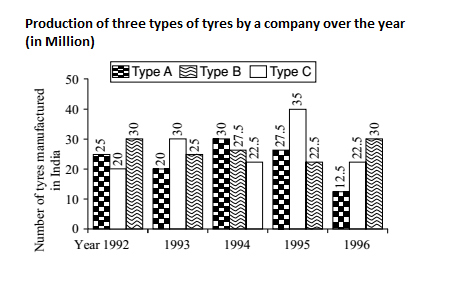## Introduction to Data Interpretation

#### Data Interpretation

Direction: Study the following graph carefully and answer the questions given below.1. What was the difference between the number of B type tyres manufactured in 1994 and 1995?

1. Required difference = Number of B type tyres in 1994 - Number of B type tyres in 1995

##### Correct Option: E

Required difference = Number of B type tyres in 1994 - Number of B type tyres in 1995
Required difference = ( 27.5 − 22.5 ) Million = 5 Million = 5000000.

1. The total number of all the three types of tyres manufactured was the least in which of the following years?

1. Only by visual observation you can find the answer. You do not need to calculate the values.

##### Correct Option: A

Only by visual observation you can find the answer. You do not need to calculate the values. I (iv) (e) Production of B type cars is more than the production of C type cars only in 1993, 1994 and 1995. We see the largest difference exists in 1995. So, the answer is 1995.

1. The total production of C type tyres in 1992 and 1993 together was what percentage of production of B type tyres in 1994?

1. Total production of C type tyres in 1992 and 1993 together = ( 30 + 25 ) = 55 Million
Production B type tyres in 1994 = 27.5 Million.

##### Correct Option: D

Total production of C type tyres in 1992 and 1993 together = ( 30 + 25 ) = 55 Million
Production B type tyres in 1994 = 27.5 Million.

 ∴ Required percentage = 55 X 100 = 200 27.5

1. What was the percentage drop in the number of C type tyres manufactured from 1993 to 1994?

1.  Required percentage drop of C type tyres = number of C type tyres from 1994 - number of C type tyres from 1993 number of C type tyres manufactured from 1993

##### Correct Option: D

 Required percentage drop of C type tyres = number of C type tyres from 1994 - number of C type tyres from 1993 number of C type tyres manufactured from 1993

 Required percentage drop of C type tyres = 30 - 27.5 X 100 = 25 % 30

1. In which of the following years was the maximum production of C type?

1. As we can see in the graph the maximum chart for the C.

##### Correct Option: E

As we can see in the graph the maximum chart for the C is in 1995.